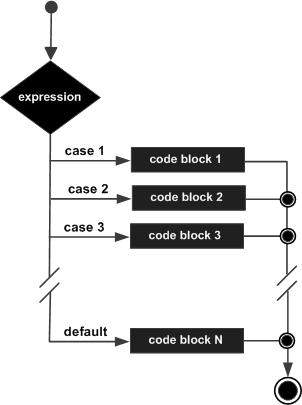# R - Switch Statement

A switch statement allows a variable to be tested for equality against a list of values. Each value is called a case, and the variable being switched on is checked for each case.

## Syntax

The basic syntax for creating a switch statement in R is −

```switch(expression, case1, case2, case3....)
```

The following rules apply to a switch statement −

• If the value of expression is not a character string it is coerced to integer.

• You can have any number of case statements within a switch. Each case is followed by the value to be compared to and a colon.

• If the value of the integer is between 1 and nargs()−1 (The max number of arguments)then the corresponding element of case condition is evaluated and the result returned.

• If expression evaluates to a character string then that string is matched (exactly) to the names of the elements.

• If there is more than one match, the first matching element is returned.

• No Default argument is available.

• In the case of no match, if there is a unnamed element of ... its value is returned. (If there is more than one such argument an error is returned.)

## Flow Diagram## Example

```x <- switch(
3,
"first",
"second",
"third",
"fourth"
)
print(x)
```

When the above code is compiled and executed, it produces the following result −

``` "third"
```
r_decision_making.htm